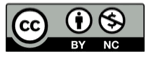# Python 中 NamedTuple 的理解

2021-12-04namedtuple（具名元组）和与 tuple 通过坐标位置辨认属性相比，可读性更好。

namedtuple（具名元组）自带属性名称，对比下面两个：

``````t1 = ('Alice', 12500, 2)
``````

namedtuple 可以使用在 tuple 的任何地方。

## 简单示例

`t` 变量指向一个新生成的类，类名叫做 T

``````>>> t=collections.namedtuple('T',["a","b","c","d"])
``````

``````>>> t1=t(a=1,b=2,c=3,d=4)
>>> print(t1)
T(a=1, b=2, c=3, d=4)
``````

``````>>> t1=t(a=1, b=2, c=3)
Traceback (most recent call last):
File "<stdin>", line 1, in <module>
TypeError: <lambda>() missing 1 required positional argument: 'd'
``````

``````>>> t1.a   # 点号表示法
1
>>> t1  # 可索引
2
>>> for item in t1: print(item)  # 可遍历
...
1
2
3
4
>>> A,B,C,D = t1   # 可拆包
>>> A
1
>>>
``````

## 工厂函数 namedtuple()

``````>>> d = dict(a=1, b=2)
>>> d
{'a': 1, 'b': 2}
``````

## namedtuple() 的参数列表

``````namedtuple(typename,
field_names,
*,
rename=False,
defaults=None,
module=None
)

``````

## 类名 typename

``````>>> import collections
>>> new_class = collections.namedtuple('aa',['a'])
>>> new_class.__class__
<class 'type'>           # 从type派生出来的
>>> new_class.__name__   # 类的名称
'aa'
``````

``````x=2
``````

``````>>> aa_object = new_class(a=1)
>>> aa_object.__class__
<class '__main__.aa'>      # 从 aa 实例化
>>> type(aa_object)
<class '__main__.aa'>      # aa 类型
>>> isinstance(aa_object,new_class)
True
>>>

``````

``````Card = collections.namedtuple('Card', ['rank', 'suit'])
``````

``````Employee = collections.namedtuple('Employee', ['name', 'city', 'salary'])
``````

``````>>> new_2_class = new_class
>>> new_2_class(a=1).__class__
<class '__main__.aa'>
>>>
``````

## 字段列表 field_names

### 字符串的序列

sequence of string 即可，比如

``````namedtuple('T',["a","b","c","d"])
namedtuple('T',("a","b","c","d"))
``````

### 空格或逗号分隔

``````namedtuple('T',"a b c d"])
namedtuple('T',"a,b,c,d")
``````

### 非法的字段名称

python 的预留关键字不能用作字段名称：

``````>>> collections.namedtuple('T',"for,b,c,d")
Traceback (most recent call last):
File "<stdin>", line 1, in <module>
File "xx.py", line 393, in namedtuple
raise ValueError('Type names and field names cannot be a '
ValueError: Type names and field names cannot be a keyword: 'for'
``````

## rename

``````>>> collections.namedtuple('T',"for,b,c,d",rename=True)._fields
('_0', 'b', 'c', 'd')

# for 被自动更名为 _0
``````

## defaults

``````>>> t=collections.namedtuple('T',"a,b,c,d")   # 没有定义默认值
>>> t()       #报错
Traceback (most recent call last):
File "<stdin>", line 1, in <module>
TypeError: <lambda>() missing 4 required positional arguments: 'a', 'b', 'c', and 'd'
>>> t=collections.namedtuple('T',"a,b,c,d",defaults=[1,2,3,4])  # 定义了默认值
>>> t()    #自动赋值
T(a=1, b=2, c=3, d=4)
>>>
``````

``````>>> t=collections.namedtuple('T',"a b c d",defaults={"a":4,"b":3,"c":2,"d":1})
>>> t()
T(a='a', b='b', c='c', d='d')
>>>
``````

``````>>> t=collections.namedtuple('T',"a b c d",defaults="1234")
>>> t()
T(a='1', b='2', c='3', d='4')
``````

### 对不上的情况

``````>>> t=collections.namedtuple('T',"a b c d",defaults="34")
``````

default 提供的数值少两个，那么实例化的时候，必然有两个参数需要用位置来赋值、

``````>>> t=collections.namedtuple('T',"a b c d",defaults="34")
>>> t(1,2)
T(a=1, b=2, c='3', d='4')
>>>
``````

## 自带函数

namedtuple 是派生自 tuple 的子类，除了继承了 tuple 的所有特性外，还自带了一些特有的方法方便使用。

### _make()

``````>>> t=collections.namedtuple('T',"a b c d")
>>> t(*[1,2,3,4])
T(a=1, b=2, c=3, d=4)
>>>
``````

### _asdict()

``````>>> t1
T(a=1, b=2, c=3, d=4)
>>> t1._asdict()
{'a': 1, 'b': 2, 'c': 3, 'd': 4}
>>>
``````

## 精彩评论